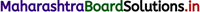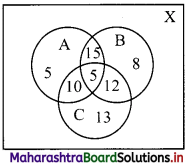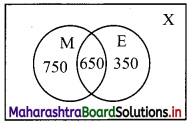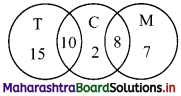# Maharashtra Board 11th Commerce Maths Solutions Chapter 1 Sets and Relations Ex 1.1

Balbharati Maharashtra State Board 11th Commerce Maths Solution Book Pdf Chapter 1 Sets and Relations Ex 1.1 Questions and Answers.

## Maharashtra State Board 11th Commerce Maths Solutions Chapter 1 Sets and Relations Ex 1.1

Question 1.
Describe the following sets in Roster form:
(i) {x / x is a letter of the word ‘MARRIAGE’}
(ii) {x / x is an integer, –$$\frac{1}{2}$$ < x < $$\frac{9}{2}$$}
(iii) {x / x = 2n, n ∈ N}
Solution:
(i) Let A = {x / x is a letter of the word ‘MARRIAGE’}
∴ A = {M, A, R, I, G, E}

(ii) Let B = {x / x is an integer, –$$\frac{1}{2}$$ < x < $$\frac{9}{2}$$}
∴ B = {0, 1, 2, 3, 4}

(iii) Let C = {x / x = 2n, n ∈ N}
∴ C = {2, 4, 6, 8, ….}

Question 2.
Describe the following sets in Set-Builder form:
(i) {0}
(ii) {0, ±1, ±2, ±3}
(iii) $$\left\{\frac{1}{2}, \frac{2}{5}, \frac{3}{10}, \frac{4}{17}, \frac{5}{26}, \frac{6}{37}, \frac{7}{50}\right\}$$
Solution:
(i) Let A = {0}
0 is a whole number but it is not a natural number.
∴ A = {x / x ∈ W, x ∉ N}

(ii) Let B = {0, ±1, ±2, ±3}
B is the set of elements which belongs to Z from -3 to 3.
∴ B = {x / x ∈ Z, -3 ≤ x ≤ 3}

(iii) Let C = $$\left\{\frac{1}{2}, \frac{2}{5}, \frac{3}{10}, \frac{4}{17}, \frac{5}{26}, \frac{6}{37}, \frac{7}{50}\right\}$$
∴ C = {x / x = $$\frac{n}{n^{2}+1}$$, n ∈ N, n ≤ 7}Question 3.
If A = {x / 6x2 + x – 15 = 0}, B = {x / 2x2 – 5x – 3 = 0}, C = {x / 2x2 – x – 3 = 0}, then find (i) (A ∪ B ∪ C) (ii) (A ∩ B ∩ C)
Solution:
A = {x / 6x2 + x – 15 = o}
∴ 6x2 + x – 15 = 0
∴ 6x2 + 10x – 9x – 15 = 0
∴ 2x(3x + 5) – 3(3x + 5) = 0
∴ (3x + 5) (2x – 3) = 0
∴ 3x + 5 = 0 or 2x – 3 = 0
∴ x = $$\frac{-5}{3}$$ or x = $$\frac{3}{2}$$
∴ A = $$\left\{\frac{-5}{3}, \frac{3}{2}\right\}$$

B = {x / 2x2 – 5x – 3 = 0}
∴ 2x2 – 5x – 3 = 0
∴ 2x2 – 6x + x – 3 = 0
∴ 2x(x – 3) + 1(x – 3) = 0
∴ (x – 3)(2x + 1) = 0
∴ x – 3 = 0 or 2x + 1 = 0
∴ x = 3 or x = $$\frac{-1}{2}$$
∴ B = {$$\frac{-1}{2}$$, 3}

C = {x / 2x2 – x – 3 = 0}
∴ 2x2 – x – 3 = 0
∴ 2x2 – 3x + 2x – 3 = 0
∴ x(2x – 3) + 1(2x – 3) = 0
∴ (2x – 3) (x + 1) = 0
∴ 2x – 3 = 0 or x + 1 = 0
∴ x = $$\frac{3}{2}$$ or x = -1
∴ C = {-1, $$\frac{3}{2}$$}

(i) A ∪ B ∪ C = $$\left\{-\frac{5}{3}, \frac{3}{2}\right\} \cup\left\{\frac{-1}{2}, 3\right\} \cup\left\{-1, \frac{3}{2}\right\}$$ = $$\left\{\frac{-5}{3},-1, \frac{-1}{2}, \frac{3}{2}, 3\right\}$$

(ii) A ∩ B ∩ C = { }

Question 4.
If A, B, C are the sets for the letters in the words ‘college’, ‘marriage’ and ‘luggage’ respectively, then verify that [A – (B ∪ C)] = [(A – B) ∩ (A – C)].
Solution:
A = {c, o, l, g, e}
B = {m, a, r, i, g, e}
C = {l, u, g, a, e}
B ∪ C = {m, a, r, i, g, e, l, u}
A – (B ∪ C) = {c, o}
A – B = {c, o, l}
A – C = {c, o}
∴ [(A – B) ∩ (A – C)] = {c, o} = A – (B ∪ C)
∴ [A – (B ∪ C)] = [(A – B) ∩ (A – C)]Question 5.
If A = {1, 2, 3, 4}, B = {3, 4, 5, 6}, C = {4, 5, 6, 7, 8} and universal set X = {1, 2, 3, 4, 5, 6, 7, 8, 9, 10}, then verify the following:
(i) A ∪ (B ∩ C) = (A ∪ B) ∩ (A ∪ C)
(ii) A ∩ (B ∪ C) = (A ∩ B) ∪ (A ∩ C)
(iii) (A ∪ B)’ = A’ ∩ B’
(iv) (A ∩ B)’ = A’ ∪ B’
(v) A = (A ∩ B) ∪ (A ∩ B’)
(vi) B = (A ∩ B) ∪ (A’ ∩ B)
(vii) n(A ∪ B) = n(A) + n(B) – n(A ∩ B)
Solution:
A = {1, 2, 3, 4}, B = {3, 4, 5, 6}, C = {4, 5, 6, 7, 8}, X = {1, 2, 3, 4, 5, 6, 7, 8, 9, 10}
(i) B ∩ C = {4, 5, 6}
∴ A ∪ (B ∩ C) = {1, 2, 3, 4, 5, 6} ……(i)
A ∪ B = {1, 2, 3, 4, 5, 6}
A ∪ C = {1, 2, 3, 4, 5, 6, 7, 8}
∴ (A ∪ B) ∩ (A ∪ C) = {1, 2, 3, 4, 5, 6} ……(ii)
From (i) and (ii), we get
A ∪ (B ∩ C) = (A ∪ B) ∩ (A ∪ C)

(ii) B ∪ C = {3, 4, 5, 6, 7, 8}
∴ A ∩ (B ∪ C) = {3, 4} …..(i)
A ∩ B = {3, 4}
A ∩ C = {4}
∴ (A ∩ B) ∪ (A ∩ C) = {3, 4} …..(ii)
From (i) and (ii), we get
A ∩ (B ∪ C) = (A ∩ B) ∪ (A ∩ C)

(iii) A ∪ B = {1, 2, 3, 4, 5, 6}
∴ (A ∪ B)’ = {7, 8, 9, 10} …….(i)
A’ = {5, 6, 7, 8, 9, 10}, B’ = {1, 2, 7, 8, 9, 10}
∴ A’ ∩ B’ = {7, 8, 9, 10} ……(ii)
From (i) and (ii), we get
(A ∪ B)’ = A’ ∩ B’

(iv) A ∩ B = {3, 4}
∴ (A ∩ B)’ = {1, 2, 5, 6, 7, 8, 9, 10} ……(i)
A’ = {5, 6, 7, 8, 9, 10}
B’ = {1, 2, 7, 8, 9, 10}
∴ A’ ∪ B’ = {1, 2, 5, 6, 7, 8, 9, 10} ……(ii)
From (i) and (ii), we get
(A ∩ B)’ = A’ ∪ B’

(v) A = {1, 2, 3, 4} …..(i)
A ∩ B = {3, 4}
B’ = {1, 2, 7, 8, 9, 10}
A ∩ B’ = {1, 2}
∴ (A ∩ B) ∪ (A ∩ B’) = {1, 2, 3, 4} ……(ii)
From (i) and (ii), we get
A = (A ∩ B) ∪ (A ∩ B’)

(vi) B = {3, 4, 5, 6} …..(i)
A ∩ B = {3, 4}
A’ = {5, 6, 7, 8, 9, 10}
A’ ∩ B = {5, 6}
∴ (A ∩ B) ∪ (A’ ∩ B) = {3, 4, 5, 6} …..(ii)
From (i) and (ii), we get
B = (A ∩ B) ∪ (A’ ∩ B)

(vii) A = {1, 2, 3, 4}, B = {3, 4, 5, 6},
A ∩ B = {3, 4}, A ∪ B = {1, 2, 3, 4, 5, 6}
∴ n(A) = 4, n(B) = 4,
n(A ∩ B) = 2,
n(A ∪ B) = 6 …..(i)
∴ n(A) + n(B) – n(A ∩ B) = 4 + 4 – 2
∴ n(A) + n(B) – n(A ∩ B) = 6 …..(ii)
From (i) and (ii), we get
n(A ∪ B) = n(A) + n(B) – n(A ∩ B)

Question 6.
If A and B are subsets of the universal set X and n(X) = 50, n(A) = 35, n(B) = 20, n(A’ ∩ B’) = 5, find
(i) n(A ∪ B)
(ii) n(A ∩ B)
(iii) n(A’ ∩ B)
(iv) n(A ∩ B’)
Solution:
n(X) = 50, n(A) = 35, n(B) = 20, n(A’ ∩ B’) = 5
(i) n(A ∪ B) = n(X) – [n(A ∪ B)’]
= n(X) – n(A’ ∩ B’)
= 50 – 5
= 45

(ii) n(A ∩ B) = n(A) + n(B) – n(A ∪ B)
= 35 + 20 – 45
= 10

(iii) n(A’ ∩ B) = n(B) – n(A ∩ B)
= 20 – 10
= 10

(iv) n(A ∩ B’) = n(A) – n(A ∩ B)
= 35 – 10
= 25Question 7.
Out of 200 students, 35 students failed in MHT-CET, 40 in AIEEE and 40 in IIT entrance, 20 failed in MHT-CET and AIEEE, 17 in AIEEE and IIT entrance, 15 in MHT-CET and IIT entrance, and 5 failed in all three examinations. Find how many students
(i) did not fail in any examination.
(ii) failed in AIEEE or IIT entrance.
Solution:
Let A = set of students who failed in MHT-CET
B = set of students who failed in AIEEE
C = set of students who failed in IIT entrance
X = set of all students
∴ n(X) = 200, n(A) = 35, n(B) = 40, n(C) = 40,
n(A ∩ B) = 20, n(B ∩ C) = 17, n(A ∩ C) = 15, n(A ∩ B ∩ C) = 5(i) n(A ∪ B ∪ C) = n(A) + n(B) + n(C) – n(A ∩ B) – n(B ∩ C) – n(A ∩ C) + n(A ∩ B ∩ C)
= 35 + 40 + 40 – 20 – 17 – 15 + 5
= 68
∴ No. of students who did not fail in any exam = n(X) – n(A ∪ B ∪ C)
= 200 – 68
= 132

(ii) No. of students who failed in AIEEE or IIT entrance = n(B ∪ C)
= n(B) + n(C) – n(B ∩ C)
= 40 + 40 – 17
= 63

Question 8.
From amongst 2000 literate individuals of a town, 70% read Marathi newspapers, 50% read English newspapers and 32.5% read both Marathi and English newspapers. Find the number of individuals who read
(i) at least one of the newspapers.
(ii) neither Marathi nor English newspaper.
(iii) only one of the newspapers.
Solution:
Let M = set of individuals who read Marathi newspapers
E = set of individuals who read English newspapers
X = set of all literate individuals
∴ n(X) = 2000,
n(M) = $$\frac{70}{100}$$ × 2000 = 1400
n(E) = $$\frac{50}{100}$$ × 2000 = 1000
n(M ∩ E) = $$\frac{32.5}{2}$$ × 2000 = 650
n(M ∪ E) = n(M) + n(E) – n(M ∩ E)
= 1400 + 1000 – 650
= 1750(i) No. of individuals who read at least one of the newspapers = n(M ∪ E) = 1750.
(ii) No. of individuals who read neither Marathi nor English newspaper = n(M’ ∩ E’)
= n(M ∪ E)’
= n(X) – n(M ∪ E)
= 2000 – 1750
= 250
(iii) No. of individuals who read only one of the newspapers = n(M ∩ E’) + n(M’ ∩ E)
= n(M ∪ E) – n(M ∩ E)
= 1750 – 650
= 1100Question 9.
In a hostel, 25 students take tea, 20 students take coffee, 15 students take milk, 10 students take both tea and coffee, 8 students take both milk and coffee. None of them take tea and milk both and everyone takes atleast one beverage, find the number of students in the hostel.
Solution:
Let T = set of students who take tea
C = set of students who take coffee
M = set of students who take milk
∴ n(T) = 25, n(C) = 20, n(M) = 15,
n(T ∩ C) = 10, n(M ∩ C) = 8, n(T ∩ M) = 0, n(T ∩ M ∩ C) = 0∴ Number of students in the hostel = n(T ∪ C ∪ M)
= n(T) + n(C) + n(M) – n(T ∩ C) – n(M ∩ C) – n(T ∩ M) + n(T ∩ M ∩ C)
= 25 + 20 + 15 – 10 – 8 – 0 + 0
= 42

Question 10.
There are 260 persons with skin disorders. If 150 had been exposed to the chemical A, 74 to the chemical B, and 36 to both chemicals A and B, find the number of persons exposed to
(i) Chemical A but not Chemical B
(ii) Chemical B but not Chemical A
(iii) Chemical A or Chemical B.
Solution:
Let A = set of persons exposed to chemical A
B = set of persons exposed to chemical B
X = set of all persons
∴ n(X) = 260, n(A) = 150, n(B) = 74, n(A ∩ B) = 36(i) No. of persons exposed to chemical A but not to chemical B = n(A ∩ B’)
= n(A) – n(A ∩ B)
= 150 – 36
= 114

(ii) No. of persons exposed to chemical B but not to chemical A = n(A’ ∩ B)
= n(B) – n(A ∩ B)
= 74 – 36
= 38

(iii) No. of persons exposed to chemical A or chemical B = n(A ∪ B)
= n(A) + n(B) – n(A ∩ B)
= 150 + 74 – 36
= 188

Question 11.
If A = {1, 2, 3}, write the set of all possible subsets of A.
Solution:
A = {1, 2, 3}
∴ { }, {1}, {2}, {3}, {1, 2}, {2, 3}, {1, 3} and {1, 2, 3} are all the possible subsets of A.Question 12.
Write the following intervals in set-builder form:
(i) (-3, 0)
(ii) [6, 12]
(iii) (6, 12)
(iv) (-23, 5)
Solution:
(i) (-3, 0) = {x / x ∈ R, -3 < x < 0}
(ii) [6, 12] = {x / x ∈ R, 6 ≤ x ≤ 12}
(iii) (6, 12) = {x / x ∈ R, 6 < x < 12}
(iv) (-23, 5) = {x / x ∈ R, -23 < x < 5}